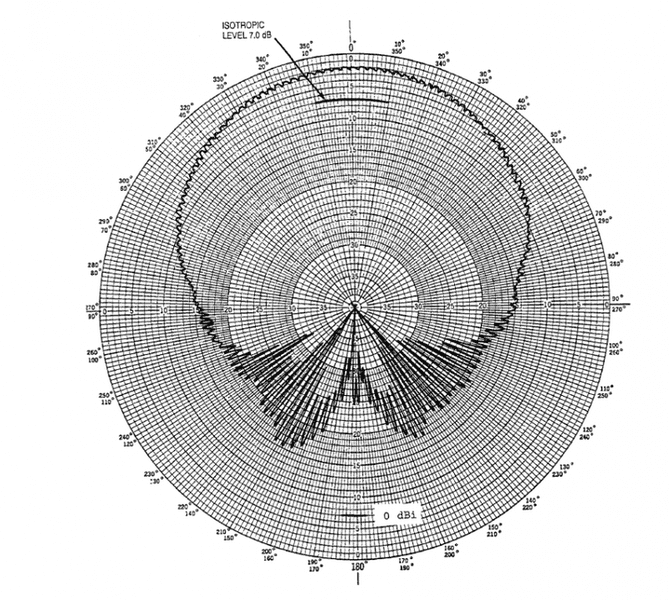# Antenna pattern scalingHello Physics Forums!

I'm not an engineer/scientist but I work with antennas and I'm having trouble deciphering the above antenna radiation pattern diagram.

I am used to seeing radiation patterns expressed in polar plots. Usually the scale is set to dBi (where the isotropic antenna = 0dBi), or it is set to main beam bore sight power (where the peak of the main beam is 0 dB). The diagram above is scaled differently.

As you can see, the isotropic level is '7 dB'. The bore sight of the main beam is at about 2 dB. The half-power points (5 dB) occur at roughly +/- 35 degrees off bore sight.

I have some questions:

1) Why is the diagram scaled this way? What does it mean to say that the bore sight of the main beam is at 2 dB? 2 dB less than what?

2) Shouldn't all of these measurements be in negative dB? e.g. shouldn't the isotropic level be -7 dB?

3) Could I go ahead and 'convert' this diagram to isotropic scale? So the bore sight is at 5 dBi, the power at 90 / 270 degrees is roughly -9 dbi, etc.?

Thanks!

#### Attachments

Baluncore
2021 Award
Welcome to PF.
1) Why is the diagram scaled this way? What does it mean to say that the bore sight of the main beam is at 2 dB? 2 dB less than what?
2 dB down from the measurement system calibration reference. Avoid “less”, use “down”.

2) Shouldn't all of these measurements be in negative dB? e.g. shouldn't the isotropic level be -7 dB?
Yes and No. It depends on if it plots gain or attenuation respectively. A gain of –3dB is an attenuation of +3dB.

3) Could I go ahead and 'convert' this diagram to isotropic scale? So the bore sight is at 5 dBi, the power at 90 / 270 degrees is roughly -9 dbi, etc.?
All things are relative. The outer circle was the reference level on the chart.
If the isotropic reference is 7dB down and the main lobe is 2dB down, then the antenna main lobe gain is 7–2 = 5 dBi.

You might want to use a reference of either dBi or dBd depending on what you need to compare it with.
Since the 0, 5, 10, 15dB … rings are in bold, the chart cannot be simply renumbered.
You must draw it again to avoid confusion later, or simply subtract the selected reference when reading the numbers.

•berkeman
marcusl